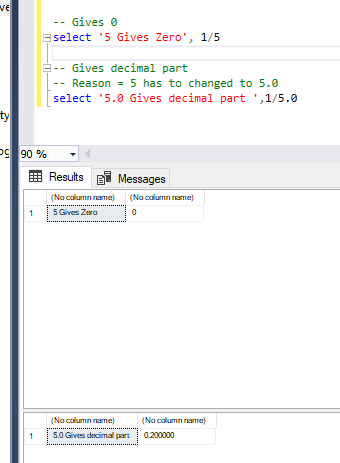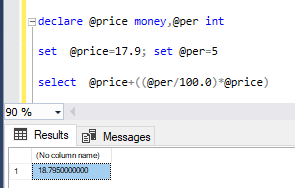Why is this returning 0?

I am expecting to be returning .05
why does this return 0
how can I get it to return .05

select cast((5/100 ) as float)100 has to be changed to 100.0

thanks
now to my next step
what am I doing wrong here
declare @price money,@per int
select @price=17.9,@per=5
select cast(@price *(@per *((@per/100.0+1))) as float)

if @per is 5 I want it to give me the price + 5 %declare @price money,@per int
set  @price=17.9; set @per=5
select  @price+((@per/100.0)*@price)

Thanks so much - this is what I needed

The reason behind this is there are two integers being used. This will produce an integer. By adding the .0 the value is changed the format to one with decimal so the answer will contain decimals.